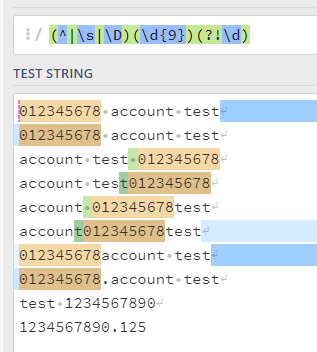# Need to pick 9 digit numeric value

Hello all,

One of my process required to pick only the 9 digit numeric value from the text. the problem is it will be as free text where there is no fixed template or position where you can look out for.

Below are the scenario’s assuming it would be
012345678 account test
012345678 account test
account test 012345678
account test012345678
account 012345678test
account012345678test
012345678account test
012345678.account test

Am able to get the true value with the regex pattern System.Text.RegularExpressions.Regex.Match(variable.Trim,“[0-9]{9}”).Value

the problem arise… when we get the value like below

test 1234567890
1234567890.125

here the value are more than 9 digit and am getting the value as true since it has 9 digit combo…

where i would require the bot not take the value at all and say true only if its find 9 digit numeric value not more than that…

Hi @Fresher

You need then specify what kind of characters you can have before and after this 9 digit group.2 Likes

Hi,

Another solution:

``````(?<=^|\D)\d{9}(?=\D|\$)
``````

Regards,

2 Likes

@alexandretperez & @Yoichi - thank you… pattern suggested by both of you worked out